This is an automatically generated documentation by LaTeX2HTML utility. In case of any issue, please, contact us at info@cfdsupport.com.

## Spalart - Allmaras model

Spalart-Allmaras model (SA) presented by Spalart and Allmaras  is an one-equational model written in terms of modified eddy viscosity. The model uses empiricism and arguments of dimensional analysis, it is independent on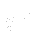, but requires the distance to the nearest wall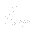. The turbulent eddy viscosity is developed with the help of model’s transport equation: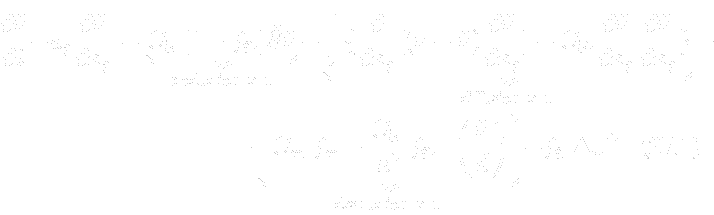where the production term is developed with the help of norm of vorticity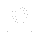. The diffusion terms are naturally connected with spatial derivatives of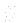. The destruction term arose from dimensional analysis.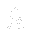and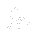are transition functions, that provide control over the laminar and turbulent regions. All the details are step by step discussed in the original article . The turbulent eddy viscosity is then:(27.29)
where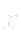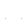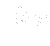is Reynolds turbulent number. Model is completed by following formulas:(27.30)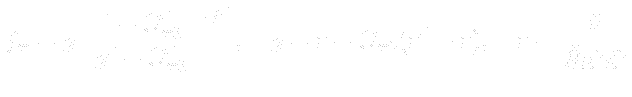(27.31)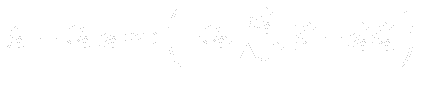(27.32)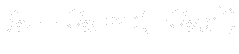(27.33)
The following table gives the model constants present in the formulas above: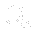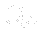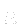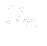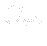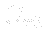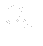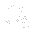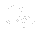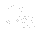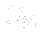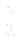0.1355 0.622 0.41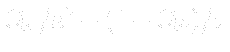0.3 2.0 7.1 1.0 2.0 1.1 2.0

Subsections